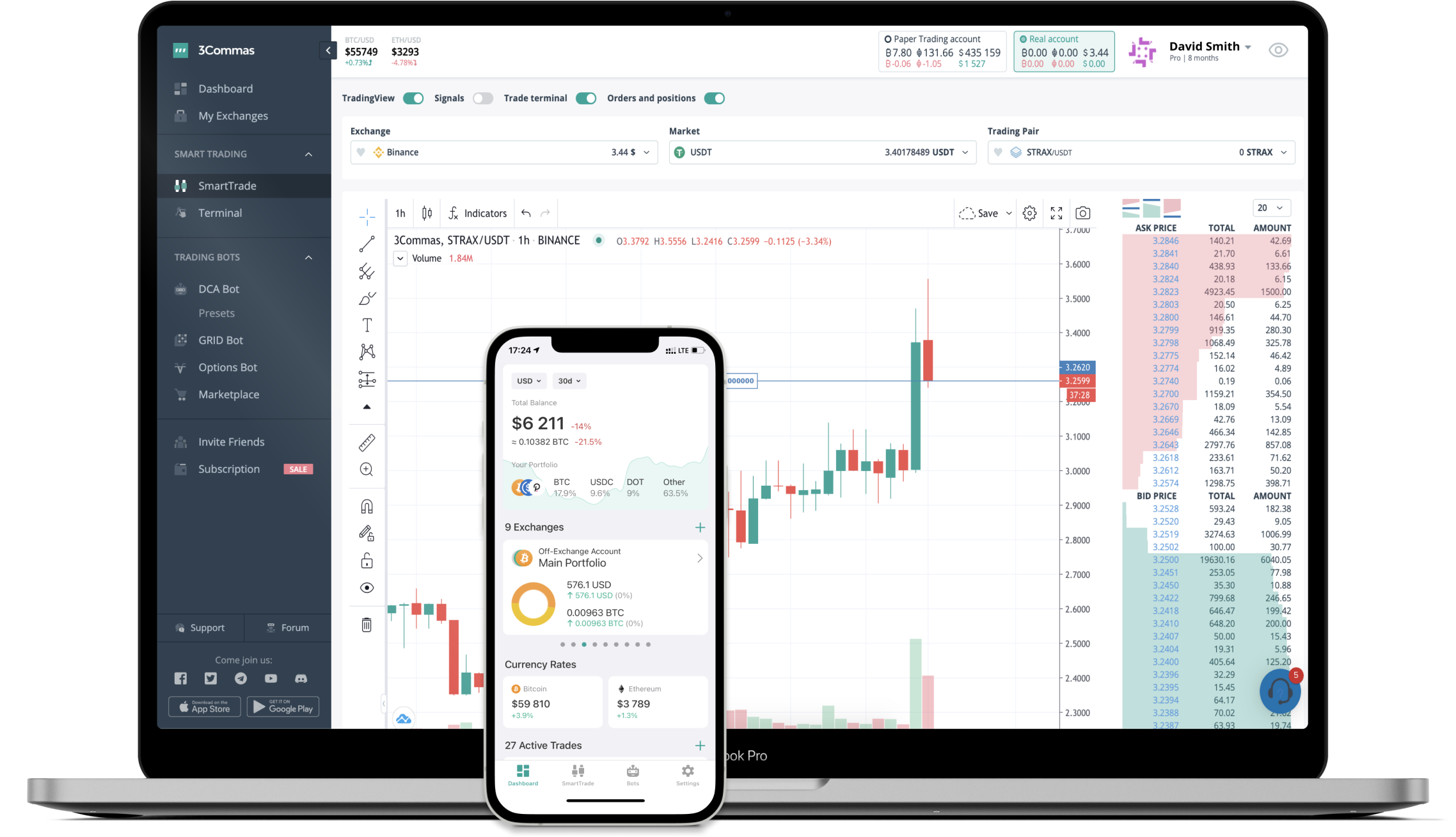# DELTA to CHF Converter

Delta Financial
PriceCHF 1.14-0.80%
VolumeCHF 6,625
How it work

The 3Commas currency calculator allows you to convert a currency from Delta Financial (DELTA) to Swiss Franc (CHF) in just a few clicks at live exchange rates.

Simply enter the amount of Delta Financial you wish to convert to CHF and the conversion amount automatically populates. You can also use our Prices Calculator Table to calculate how much your currency is worth in other denominations, i.e. .1 DELTA, .5 DELTA, 1 DELTA, 5 DELTA, or even 10 DELTA.

## DELTA to CHF Exchange Calculator

The chart displays Delta Financial price changes in CHF.

The ultimate tools for crypto traders to maximize trading profits while minimizing risk and loss.## Latest DELTA to CHF price calculator

Delta Financial market price is updated every three minutes and is automatically displayed in CHF. Below are the most popular denominations to convert to CHF.

Delta Financial
Swiss Franc
0.01
CHF
0.11
CHF
1.14
CHF
2.28
CHF
3.42
CHF
5.70
CHF
11.40
CHF
28.50
CHF
57.00
CHF
114.00
CHF
285.00
CHF
570.00
CHF
1140.00
CHF
2850.00
CHF
Swiss Franc
Delta Financial
0.00877193
DELTA
0.08771930
DELTA
0.87719298
DELTA
1.75438596
DELTA
2.63157895
DELTA
4.38596491
DELTA
8.77192982
DELTA
21.92982456
DELTA
43.85964912
DELTA
87.71929825
DELTA
219.29824561
DELTA
438.59649123
DELTA
877.19298246
DELTA
2192.98245614
DELTA

## FAQ

### How much is 1 Delta Financial in Swiss Franc?

Delta Financial price in CHF is constantly changing.

At this moment, 1 Delta Financial equals 1.14 CHF

### How do I calculate the conversion from DELTA to CHF?

The 3Commas Delta Financial Calculator allows you to easily calculate the conversion price of DELTA to CHF by simply entering the amount of Delta Financial in the corresponding field and will automatically convert the value in Swiss Franc (CHF).

You can also use our Delta Financial price table above to check the latest Delta Financial price in major fiat and crypto currencies.

### How can I convert Delta Financial to CHF?

The most common way of converting DELTA to CHF is by using a Crypto Exchange or a P2P (person-to-person) exchange platform like LocalBitcoins, etc.